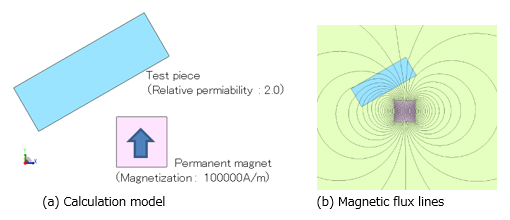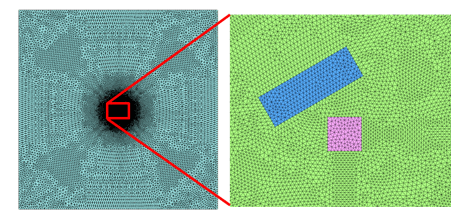## [W-SE-74] Electromagnetic Force Calculations Using the Adaptive Function

Contents
1. Introduction
2. Several Methods for Improving Electromagnetic Force Accuracy
3. Factors That Cause Electromagnetic Force Measurement Errors
4. Electromagnetic Force Calculation Using Adaptive Analysis
5. Conclusion
6. References

### 1. Introduction

One of the motivations for performing electromagnetic field analysis in electrical equipment design is the evaluation of electromagnetic force. However, when a disturbance is added which slightly jumbles the main magnetic flux spreading into the air gap, sometimes the electromagnetic forces cannot reach equilibrium. The model of the magnet and test piece in Fig. 1 is a typical example. The field made by magnets with a magnetization of 100,000 A/m dominates the space in which the test piece with a relative magnetic permeability of 2.0 slightly jumbles the mail field. Using the mesh in Fig. 2, the measurement error between the electromagnetic force acting on the magnet and the electromagnetic force acting on the test piece is calculated to be 51.5%. Here, the measurement error of the electromagnetic force is calculated as a relative value by dividing the absolute value of electromagnetic force acting on the test piece by the absolute value of the difference between the electromagnetic force acting on the test piece and that of the magnet. Despite being a simple 2D problem calculated with a mesh having 51,472 elements, it is a troublesome problem because the measurement error gets to be 50% or more.
In this report, we clarify the causes of measurement errors for electromagnetic force as well as show that adaptive analysis can be the way to solve this issue. As a calculation method for electromagnetic force, there is the surface force method and the nodal force method. However, since they are both essentially the same, we will proceed with the nodal force method in this example.Fig. 1 Magnet and test piece modelsFig. 2 Mesh diagram (number of elements: 51,472)
Even with a resolution of 51,472 elements, an error of 51.5% occurs in the equilibrium of electromagnetic force.

### 2. Several Methods for Improving Electromagnetic Force Accuracy

In FEM, generally, higher mesh resolutions produce improved calculation accuracies. That is why making finer meshes is one of the first improvement methods that people think of. Fig. 2 is a standard display of the mesh, Fig. 3(a)—Fine mesh A—is a mesh diagram for when mesh is made uniformly fine, and Fig. 3(b)—Fine mesh B—is mesh uniformly made even more fine. The number of elements in Fine mesh A is 130,376, and there is an 8.2% measurement error of electromagnetic force when this mesh is used. The number of elements in Fine mesh B is 444, 818 and there is a 6.4% error of electromagnetic force when using this mesh. Despite the fact that both mesh element counts increased, an error of 5% or more still remains.

Protected content here, for members only.
You need to sign in as a JMAG software regular user (paid membership) or JMAG WEB MEMBER (free membership).

By registering as a JMAG WEB MEMBER, you can browse technical materials and other member-only contents for free.
If you are not registered, click the “Create an Account” button.

Remember me